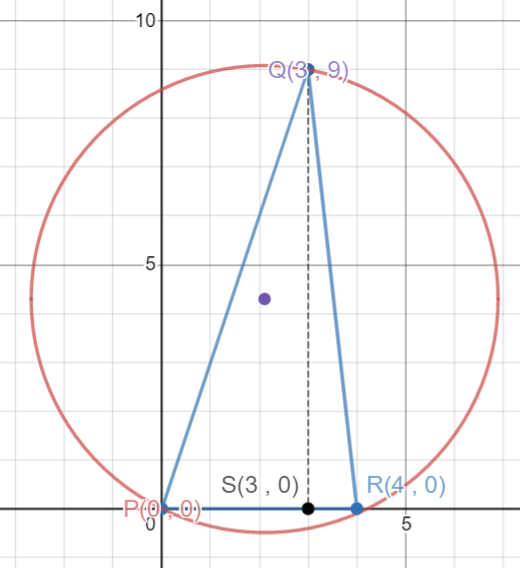# CAT 2020 Question Paper | Quants Slot 3

###### CAT Previous Year Paper | CAT Quants Questions | Question 19

This question is from Co-ordinate Geometry. Questions that appear in the CAT Exam from Co-ordinate Geometry tests an aspirant on the graphical representation Geometrical shapes, Distance between points, Section formula, Intercepts, Circles, and so on. In CAT Exam, one can generally expect to get approx. 1 question Coordinate Geometry. Make sure to a get good hold on this topic during your CAT Preparation. Practice CAT Question Paper from 2IIM CAT Question Bank and get a kick-ass CAT score.

Question 19 : The vertices of a triangle are (0,0), (4,0) and (3,9). The area of the circle passing through these three points is

1. $$frac{14π}{3}$ 2. $\frac{123π}{7}$ 3. $\frac{205π}{9}$ 4. $\frac{12π}{5}$ ## Best CAT Online Coaching Try upto 40 hours for free Learn from the best! #### 2IIM : Best Online CAT Coaching. ### Video Explanation ## Best CAT Coaching in Chennai #### CAT Coaching in Chennai - CAT 2022Limited Seats Available - Register Now! ### Explanatory Answer A circle is circumscribed on the points$0,0) (4,0) and (3,9)
We are asked to find the area of this circle

We know that the area of a triangle with sides a, b and c, inscribed ina circle of radius R is given by
Area = $$frac{abc}{4R}$With the help of teh co-ordinates of the triangle it is easy to find the height of the triangle, which is QS = 9 units. Hence the area of the triangle = A = $\frac{1}{2}$ × base × height = $\frac{1}{2}$ × PR × QS = $\frac{1}{2}$ × 4 × 9 = 18 sq. units a = PR = 4 units b = QR = √$QS2 + SR2) = √(92 + 12) = √82
c = PQ = √(PS2 + PQ2) = √(32 + 92) = √90

W.K.T Area = $$frac{abc}{4R}$ 18 = $\frac{4 × √82 × √90}{4R}$ R = $\frac{√205}{3}$ Now that we know the radius of the circle the area of the circle = π × R2 = π × $\frac{√205}{3}$ × $\frac{√205}{3}$ = π × $\frac{205}{9}$ = $\frac{205π}{9}$ The question is "The vertices of a triangle are$0,0), (4,0) and (3,9). The area of the circle passing through these three points is"

##### Hence, the answer is, "$$frac{205π}{9}$" ###### Best CAT Online Coaching Try upto 40 hours for free Learn from the best! ###### Prepare for CAT 2023 with 2IIM's Daily Preparation Schedule ###### Know all about CAT Exam Syllabus and what to expect in CAT ###### Already have an Account? ###### CAT Coaching in ChennaiCAT 2023 Classroom Batches Starting Now! @Gopalapuram ###### Best CAT Coaching in Chennai Introductory offer of 5000/- Attend a Demo Class ###### Best Indore IPM & Rohtak IPM CoachingSignup and sample 9 full classes for free. Register now! ##### Where is 2IIM located? 2IIM Online CAT Coaching A Fermat Education Initiative, 58/16, Indira Gandhi Street, Kaveri Rangan Nagar, Saligramam, Chennai 600 093 ##### How to reach 2IIM? Mobile:$91) 99626 48484 / 94459 38484
WhatsApp: WhatsApp Now
Email: info@2iim.com